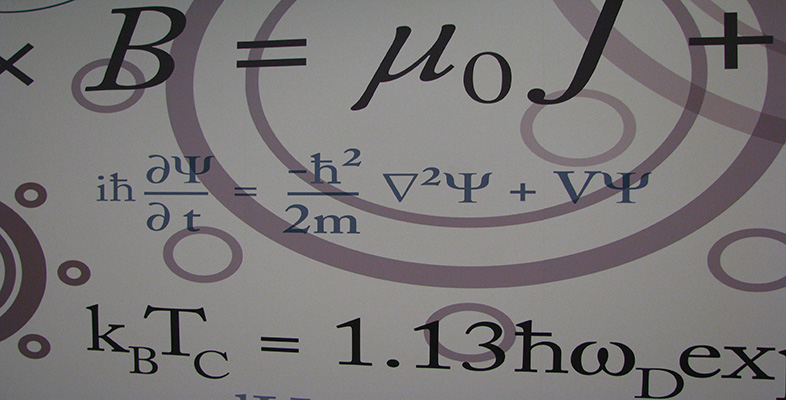Number systems

This free course is available to start right now. Review the full course description and key learning outcomes and create an account and enrol if you want a free statement of participation.

Free course

3.3 Operations in modular arithmetic

The Division Algorithm tells us that all the possible remainders on division by an integer n lie in the set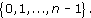We denote this set by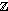n. For each integer n ≥ 2 we have a setn, and it is on these sets that we perform modular arithmetic. The modular addition operations +n and modular multiplication operations ×n are defined as follows.

Definitions

For any integer n ≥ 2,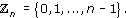For a and b inn, the operations +n and ×n are defined by:

• a +nb is the remainder of a + b on division by n;

• a ×nb is the remainder of a × b on division by n.

The integer n is called the modulus for this arithmetic.

Note: a +nb is read as a plus b (mod n), and may also be written a + b (mod n). Similarly, a ×nb is read as a times b (mod n) and may also be written as a × b (mod n).

For example,7 = { 0,1,2,3,4,5,6} and we have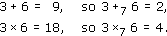You have certainly met some modular arithmetic before, as the operations +12 and +24 are used in measuring time on 12-hour and 24-hour clocks, respectively.

Exercise 41

Evaluate the following.

• (a)  3 +5 7,     4 +17 5,     8 +16 12.

• (b)  3 ×5 7,     4 ×17 5,     8 ×16 12.

Solution

• (a)  3 +5 7 = 0,     4 +17 5 = 9,     8 +16 12 = 4.

• (b)  3 ×5 7 = 1,     4 ×17 5 = 3,     8 ×16 12 = 0.

In Sections 1 and 2 we listed some properties satisfied by the real and complex numbers. We now investigate whether the setsn satisfy similar properties.

We also investigate what equations we can solve inn; for example, can we solve the equations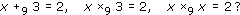These may look much simpler than the equations that we were trying to solve in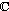, but they pose interesting questions. We shall see that the answers may depend on the modulus that we are using.

Before we discuss these questions further, we look at addition and multiplication tables, which provide a convenient way of studying addition and multiplication inn.

We consider addition first. Here are the addition tables for4 and7.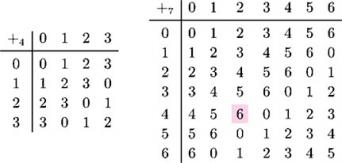In order to evaluate 4 +7 2, say, we look in the row labelled 4 and the column labelled 2 in the second table to obtain the answer 6.

Exercise 42

• (a)  Use the tables above to solve the following equations.

• (i)  x +4 3 = 2

• (ii)  x +7 5 = 2

• (iii)  x +4 2 = 0

• (iv)  x +7 5 = 0

• (b)  What patterns do you notice in the tables?

Solution

• (a)

• (i)  3 +4 3 = 2, so x = 3.

• (ii)  4 +7 5 = 2, so x = 4.

• (iii)  2 +4 2 = 0, so x = 2.

• (iv)  2 +7 5 = 0, so x = 2.

• (b)  You may have noticed that:

1. each element appears exactly once in each row and exactly once in each column;

2. there is a pattern of diagonal stripes running down from right to left.

Exercise 43

• (a)  Construct the addition table for6.

• (b)  Solve the equations x +6 1 = 5 and x +6 5 = 1.

Solution

• (a)

•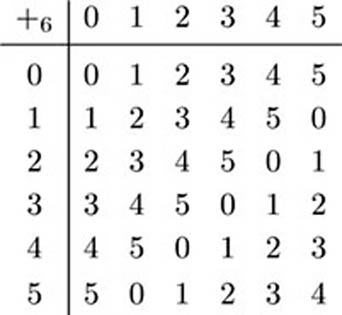• (b)  x +6 1 = 5 has solution x = 4.

• x +6 5 = 1 has solution x = 2.

For every integer n ≥ 2, the additive properties ofn are the same as the additive properties of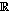, as follows.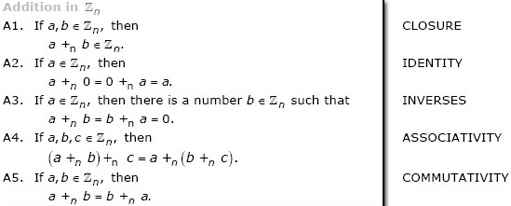Property A1 follows from the Division Algorithm and the definition ofn. The other properties can be deduced from the corresponding properties for integers.

Exercise 44

By using the corresponding property for integers, prove property A5.

Solution

By definition, a +nb and b +na are the remainders of a + b and b + a, respectively, on division by n. But a + b = b + a, so a +nb = b+na.

If a, b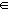n and a +nb = 0, then we say that b is the additive inverse of a inn. For example, 4 and 5 belong to9 and 4 +9 5 = 0, so 5 is the additive inverse of 4 in9. Property A3 states that every element ofn has an additive inverse inn.

Additive inverses are sometimes written in the form −na; that is, if a +nb = 0, then we write b = −na. For example, 5 = −94.

Exercise 45

• (a)  Use the addition table for7 (which appear above Exercise 42) to complete the following table of additive inverses in7.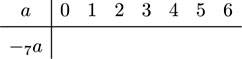• (b)  Complete the following table of additive inverses inn, explaining why your answers are correct.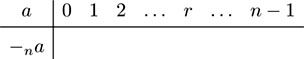Solution

• (a)

•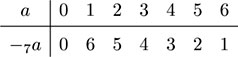• (b)

•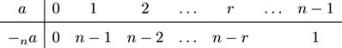• The additive inverse of 0 is always 0, since 0 +n 0 = 0. For any integer r > 0 inn, nrn and r + (nr) = n, so r +n (nr) = 0.

The existence of additive inverses means that, as well as doing addition modulo n, we can also do subtraction. We define anb or, equivalently, ab (mod n), to be the remainder of ab on division by n.

(With this definition, anb is equal to a +n (−nb).)

For example, to find 2 −8 7, we have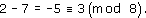Since 38, it follows that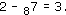M208_6